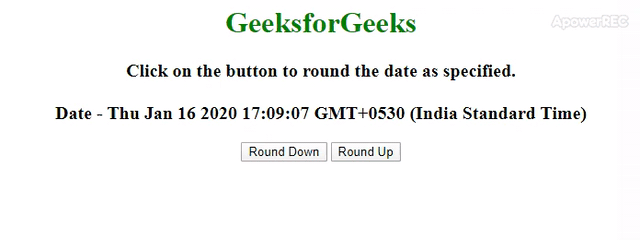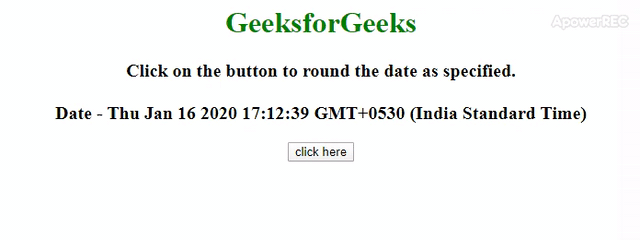Related Articles

# How to Round off Time to Nearest 5 Min using JavaScript ?

• Last Updated : 29 Jan, 2020

Given a JavaScript date and the task is to round it to 5 minutes with the help of JavaScript. There are two approaches that are discussed below:

Approach 1: In this approach, both options are available to either round down or round up the date object. This example uses basic Math.floor() function and Math.ceil() function to perform the operation.

• Example: This example implements the above approach.
 ```<``html``>`` ` `<``head``>``    ``<``title``>``        ``Round off a Date Object to 5``        ``minutes in JavaScript.``    `````` ` `<``body` `style``=``"text-align:center;"``>``     ` `    ``<``h1` `style``=``"color:green;"``>``        ``GeeksforGeeks``    ````     ` `    ``<``p` `id``=``"gfg"` `style="font-size: 20px; ``                    ``font-weight: bold">``    ````     ` `    ``<``button` `onclick``=``"GFG_Fun1();"``>``        ``Round Down``    ````     ` `    ``<``button` `onclick``=``"GFG_Fun2();"``>``        ``Round Up``    ````     ` `    ``<``p` `id``=``"geeks"` `style="font-size: 26px; ``            ``font-weight: bold;color: green;">``    ````     ` `    ``<``script``>``        ``var up = document.getElementById('gfg');``        ``var down = document.getElementById('geeks');``        ``var date = new Date();``        ``up.innerHTML = "Click on the button to "``                ``+ "round the date as specified."``                ``+ "<``br``><``br``>Date - " + date;`` ` `        ``function GFG_Fun1() {``             ` `            ``// ms in 5 minutes.``            ``var coff = 1000 * 60 * 5; ``             ` `            ``down.innerHTML = new Date(``                    ``Math.floor(date / coff) * coff);``        ``}`` ` `        ``function GFG_Fun2() {``             ` `            ``// ms in 5 minutes.``            ``var coff = 1000 * 60 * 5; ``            ``down.innerHTML = new Date(``                    ``Math.ceil(date / coff) * coff);``        ``}``    `````` ` ``
• Output:Approach 2: This example uses basic Math.round() function to perform the operation. Calculate the milliseconds in 5 minutes, divide the date object by milliseconds and get the round value then again multiply the milliseconds.

• Example: This example implements the above approach.
 ```<``html``>`` ` `<``head``>``    ``<``title``>``        ``Round off a Date Object to 5``        ``minutes in JavaScript.``    `````` ` `<``body` `style``=``"text-align:center;"``>``     ` `    ``<``h1` `style``=``"color:green;"``>``        ``GeeksforGeeks``    ````     ` `    ``<``p` `id``=``"GFG_UP"` `style` `=``        ``"font-size: 20px;font-weight: bold"``> ``    ````     ` `    ``<``button` `onclick` `= ``"GFG_Fun();"``> ``        ``click here ``    `` ``     ` `    ``<``p` `id``=``"GFG_DOWN"` `style = "font-size: 26px; ``            ``font-weight: bold;color: green;"> ``    ````     ` `    ``<``script``> ``        ``var up = document.getElementById('GFG_UP');``        ``var down = document.getElementById('GFG_DOWN');``        ``var date = new Date();``        ``up.innerHTML = "Click on the button to "``                ``+ "round the date as specified."``                ``+ "<``br``><``br``>Date - " + date;``         ` `        ``function GFG_Fun() {``             ` `            ``// ms in 5 minutes.``            ``var coff = 1000 * 60 * 5; ``            ``down.innerHTML = new Date(Math.round(``                    ``date.getTime() / coff) * coff);``        ``} ``    `` ```` ` `                           `
• Output:Hey geek! The constant emerging technologies in the world of web development always keeps the excitement for this subject through the roof. But before you tackle the big projects, we suggest you start by learning the basics. Kickstart your web development journey by learning JS concepts with our JavaScript Course. Now at it’s lowest price ever!

My Personal Notes arrow_drop_up